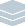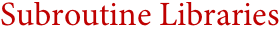Wolfram Archive

# Subroutine Libraries(NAG, IMSL, LAPACK, GSL, IT++, ...)
Created in the 1960s as an add-on to languages like Fortran, subroutine libraries were a common feature of early technical computing. But the release of Mathematica in 1988 ushered in a new era, in which technical computing could immediately be done in a single unified system, without having to assemble collections of subroutines.
After over two decades of growth, Mathematica now contains by far the world's largest integrated web of algorithms—including efficient versions of essentially every corner of functionality found in every standard subroutine library. And through new generations of algorithms made possible by its immediate ability to call on symbolic, geometric, discrete and other methods, Mathematica's algorithms routinely outperform the traditional algorithms found in subroutine libraries.
Mathematica dramatically simplifies access to sophisticated numerical methods—not only by providing an integrated interactive environment, but also through its unique methodology of automatic algorithm selection, which robustly selects optimal methods for particular problems, while allowing experts to choose specific named methods.
Mathematica Features Typical of Subroutine Libraries:
Key Advantages of Mathematica Compared to Subroutine Libraries:
Interoperability with Subroutine Libraries:
Interesting Tidbits:
• Functions like NDSolve are equivalent to hundreds of routines in multiple subroutine libraries
• Mathematica internally uses routines from many top subroutine libraries
• Mathematica is usually the first computational system to run on new hardware platforms
• Many algorithms in Mathematica are now found with Wolfram Research's unique automated algorithm discovery methods